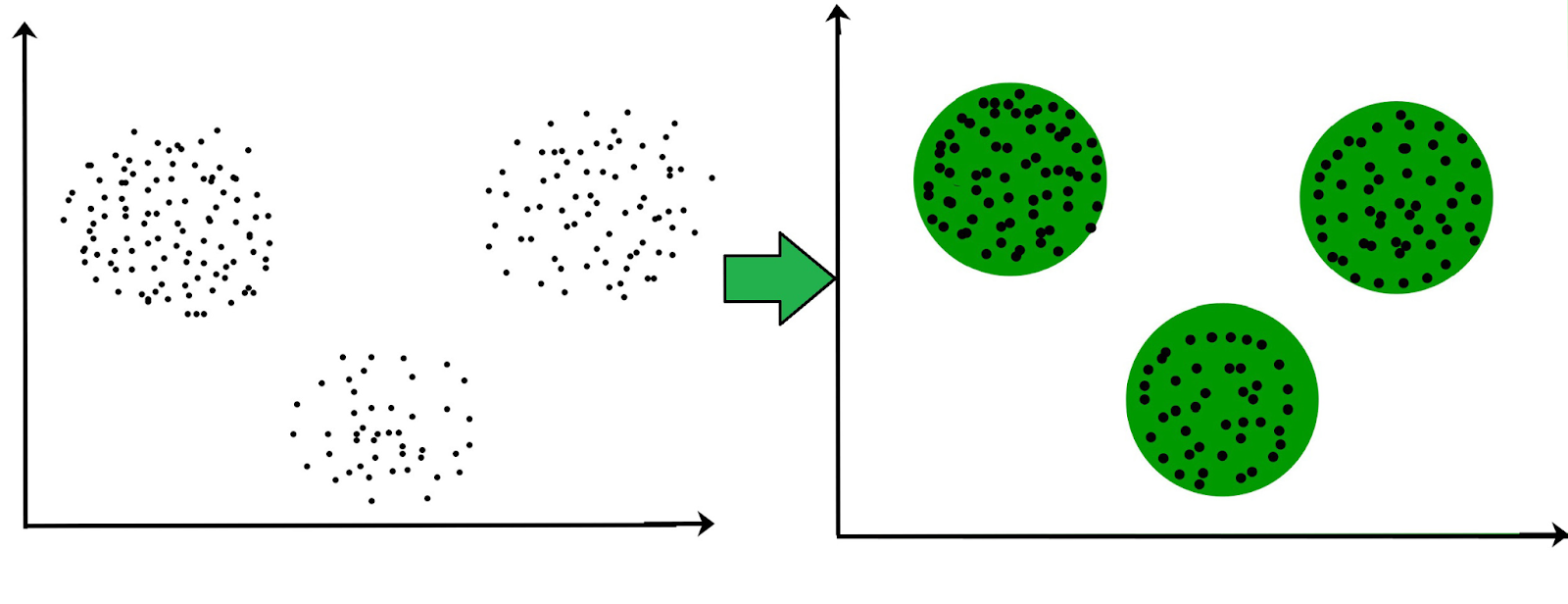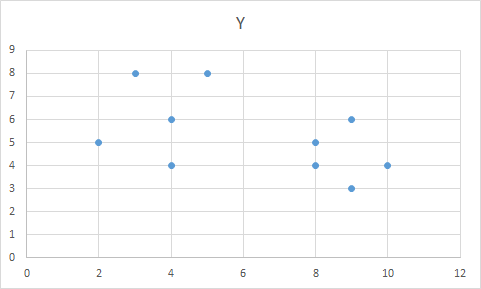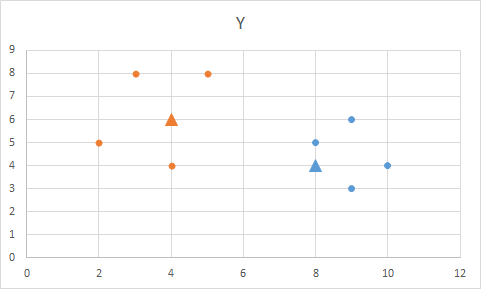# K-Medoids Algorithm

## Introduction

What is Clustering?

Clustering is a technique that divides our data sample into groups(or clusters). The most similar points are closer to each other, and there is a considerable distance between dissimilar points. It is a technique used in unsupervised algorithms to label our unlabelled data.Source: GeeksForGeeks

For example, the data in the above image could represent the behavior patterns(such as the amount of money spent, No. of items bought, etc.) of customers. As we can see, we can divide the data into three clusters, i.e., three customer segments.

In this blog, we will understand what the K medoids algorithm is, see one example of how the algorithm works, and how to code the algorithm in Python.

## K-Medoids Algorithm

K-Medoids is an unsupervised clustering algorithm in which data points called “medoids" act as the cluster's center. A mediod is a point in the cluster whose sum of distances(also called dissimilarity) to all the objects in the cluster is minimal. The distance can be the Euclidean distance, Manhattan distance, or any other suitable distance function.

Therefore, the K -medoids algorithm divides the data into K clusters by selecting K medoids from our data sample.

Working of the Algorithm

The steps taken by the K-medoids algorithm for clustering can be explained as follows:-

1. Randomly select k points from the data( k is the number of clusters to be formed). These k points would act as our initial medoids.
2. The distances between the medoid points and the non-medoid points are calculated, and each point is assigned to the cluster of its nearest medoid.
3. Calculate the cost as the total sum of the distances(also called dissimilarities) of the data points from the assigned medoid.
4. Swap one medoid point with a non-medoid point(from the same cluster as the medoid point) and recalculate the cost.
5. If the calculated cost with the new medoid point is more than the previous cost, we undo the swap, and the algorithm converges else; we repeat step 4

Finally, we will have k medoid points with their clusters.

Let’s understand the working of the algorithm with the help of an example.

For the below example, I will be using Manhattan Distance as the distance metric for calculating the distance between the points.

Manhattan Distance between two points (x1,y1) and (x2,y2) is given as

Mdist= |x2-x1| + |y2-y1|

Example

Let's suppose we have the data given below, and we want to divide the data into two clusters, i.e., k=2.

If we plot the data, it looks like the below image.For step 1, let's pick up two random medoids(as our k=2). So we pick M1 (8,4) and M2 (4,6) as our initial medoids.

Let’s calculate the distance of each data point from both the medoids.

Each point is assigned to the cluster of that medoid whose distance is less.

Points (1,2,7,10) are assigned to M1(8,4) and points (3,4,5,6) are assigned to M2(4,6)

Therefore,

Cost = (3+2+1+2)+(2+3+3+3)

= (8)+(11)

= 19

Now let us randomly select another medoid and swap it.  Let us check by having M1 as (8,5).

The new medoids are M1(8,5) and M2(4,6)

Therefore distance of each point from M1(8,5) and M2(4,6) is calculated as follows:-

Points (1,2,7,10) are assigned to M1(8,5) and points (3,4,5,6) are assigned to M2(4,6)

New Total Cost= (2+3+1+3)+(2+3+3+3)

= (9)+(11)

=  20

Now let’s calculate the Swap Cost

Swap Cost= New Total Cost- Cost

= 20-19

= 1

Since Swap Cost>0, we would undo the swap.

Our final medoids are M1(8,4) and M2(4,6), and the two clusters are formed with these medoids.

The orange dots represent the first cluster, and the blue dots represent the second cluster. The triangles represent the medoids of the clusters.## Python Implementation of K-Medoids Algorithm

Firstly, we need to install scikit-learn-extra, an extension of scikit-learn designed to implement more advanced algorithms.

``!pip install scikit-learn-extra``

Let's import the necessary libraries.

``````from sklearn_extra.cluster import KMedoids
import numpy as np``````

We will use the data created above for this implementation. Therefore let’s create an array of the data.

``data=np.asarray([[9,6],[10,4],[4,4],[5,8],[3,8],[2,5],[8,5],[4,6],[8,4],[9,3]])``

Now we use KMedoids from scikit-learn-extra to fit the data to the K-Medoid algorithm.

``kmedoids = KMedoids(n_clusters=2).fit(data)``

We can see the labels of each point via the below command.

``kmedoids.labels_``

Label 0 represents the first cluster, and label 1 represents the second cluster. The output is given below.

``````array([[4, 6],
[8, 4]])``````

We can also see the final medoids with the command below.

``kmedoids.cluster_centers_``

The final output of the medoids is given as:-

``````array([[4, 6],
[8, 4]])``````

Hence, the final two medoids are the same as what we computed above.

1. What is the difference between K-means and K-medoids clustering?
1. K-means selects the average of all the cluster points as its center( which may or may not be one of the data points), while K-medoids always pick an actual data point as the cluster's center.
2. K- means attempts to minimize the total squared error. In contrast, K-medoids attempts to minimize the sum of dissimilarities between the points labeled in the cluster and the cluster's center.

2. What are the advantages of the K-medoids algorithm?
1. K- medoids algorithm is robust to outliers and noise as the medoids are the most central data point of the cluster, such that its distance from other points is minimal.
2. K-Medoids algorithm can be used with arbitrarily chosen dissimilarity measures (e.g., cosine similarity) or any distance metric.

3. What are the drawbacks of the K-medoids algorithm?
1. The K-medoids algorithm has large time complexity. Therefore, it works efficiently for small datasets but doesn't scale well for large datasets.
2. The K-Medoid algorithm is not suitable for clustering non-spherical (arbitrary-shaped) groups of objects.

## Key Takeaways

In this blog, we understood the working of the K-Medoids algorithm along with an example and implemented the algorithm in python.

K-Medoids is a powerful unsupervised clustering algorithm that is robust to outliers and works efficiently for small datasets.

I hope this blog gave you a complete understanding of the algorithm and provided sufficient knowledge to implement the algorithm for practical use cases.